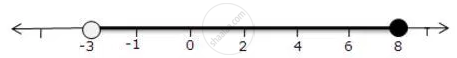Share

Represent the Following Inequalities on Real Number Lines 8 >= X >-3 - ICSE Class 10 - Mathematics

ConceptLinear Inequations in One Unknown

Question

Represent the following inequalities on real number lines

8 >= x > -3

Solution

8 >= x > -3

Solution on number line isIs there an error in this question or solution?

APPEARS IN

Solution Represent the Following Inequalities on Real Number Lines 8 >= X >-3 Concept: Linear Inequations in One Unknown.
S